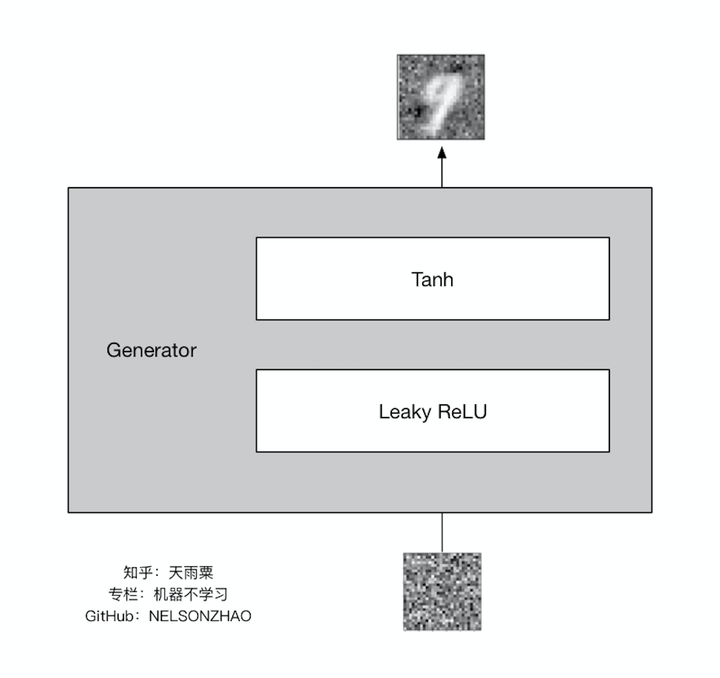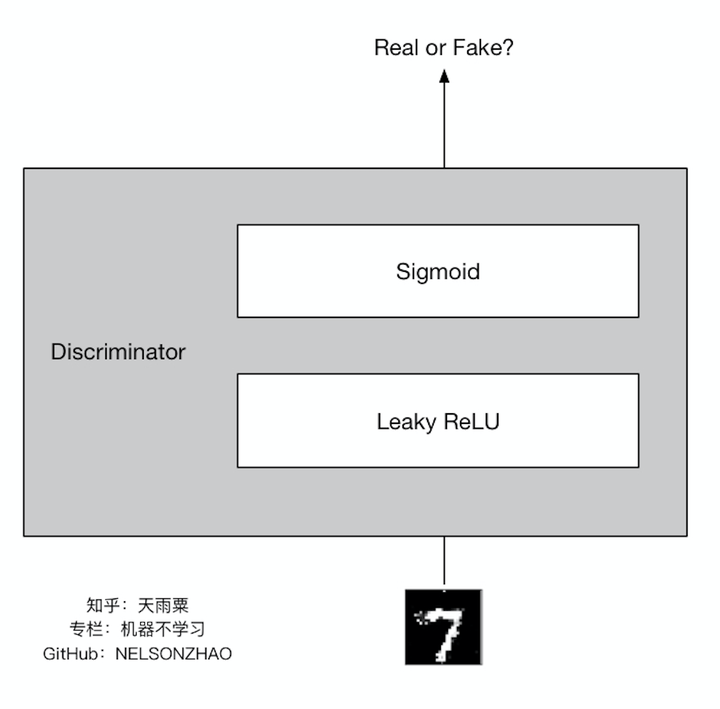前言什么是GAN• 对于给定的真实图片（real image），判别器要为其打上标签1；
• 对于给定的生成图片（fake image），判别器要为其打上标签0；
• 对于生成器传给辨别器的生成图片，生成器希望辨别器打上标签1。

代码运行环境

• TensorFlow 1.0
• Python 3
• Github地址 Github
需要说明的是，这个代码并非我所写，而是我在Github上面找到的，而且需要修改几处小的地方才能完整的跑起来，这个我后面会提到。

代码分析

1.参数定义

```class MnistModel:

def __init__(self):
# mnist测试集
# 图片大小
self.img_size = self.mnist.train.images.shape
# 每步训练使用图片数量
self.batch_size = 64
# 图片分块数量
self.chunk_size = self.mnist.train.num_examples // self.batch_size
# 训练循环次数
self.epoch_size = 300
# 抽取样本数
self.sample_size = 25
# 生成器判别器隐含层数量
self.units_size = 128
# 学习率
self.learning_rate = 0.001
# 平滑参数
self.smooth = 0.1
```

2.真实图片与混淆图片

```        import os   # 不加这一句博客的网页不能识别代码类型，不会高亮显示

# 真实图片与混淆图片
# 不确定输入图片数量 用None
real_imgs = tf.placeholder(tf.float32, [None, self.img_size], name='real_imgs')
fake_imgs = tf.placeholder(tf.float32, [None, self.img_size], name='fake_imgs')
```

3.生成器

```    import os   # 不加这一句博客的网页不能识别代码类型，不会高亮显示

def generator_graph(fake_imgs, units_size, out_size, alpha=0.01):
# 生成器与判别器属于两个网络 定义不同scope
with tf.variable_scope('generator'):
# 构建一个全连接层
layer = tf.layers.dense(fake_imgs, units_size)
# leaky ReLU 激活函数
relu = tf.maximum(alpha * layer, layer)
# dropout 防止过拟合
drop = tf.layers.dropout(relu, rate=0.2)
# logits
# out_size应为真实图片size大小
logits = tf.layers.dense(drop, out_size)
# 激活函数 将向量值限定在某个区间 与 真实图片向量类似
# 这里tanh的效果比sigmoid好一些
# 输出范围(-1, 1) 采用sigmoid则为[0, 1]
outputs = tf.tanh(logits)
return logits, outputs
```4.判别器

```    import os   # 不加这一句博客的网页不能识别代码类型，不会高亮显示

def discriminator_graph(imgs, units_size, alpha=0.01, reuse=False):
with tf.variable_scope('discriminator', reuse=reuse):
# 构建全连接层
layer = tf.layers.dense(imgs, units_size)
# leaky ReLU 激活函数
relu = tf.maximum(alpha * layer, layer)
# logits
# 判断图片真假 out_size直接限定为1
logits = tf.layers.dense(relu, 1)
# 激活函数
outputs = tf.sigmoid(logits)
return logits, outputs
```5.损失

```    import os   # 不加这一句博客的网页不能识别代码类型，不会高亮显示

def loss_graph(real_logits, fake_logits, smooth):
# 生成器图片loss
# 生成器希望判别器判断出来的标签为1
gen_loss = tf.reduce_mean(tf.nn.sigmoid_cross_entropy_with_logits(logits=fake_logits, labels=tf.ones_like(fake_logits) * (1 - smooth)))
# 判别器识别生成器图片loss
# 判别器希望识别出来的标签为0
fake_loss = tf.reduce_mean(tf.nn.sigmoid_cross_entropy_with_logits(logits=fake_logits, labels=tf.zeros_like(fake_logits)))
# 判别器识别真实图片loss
# 判别器希望识别出来的标签为1
real_loss = tf.reduce_mean(tf.nn.sigmoid_cross_entropy_with_logits(logits=real_logits, labels=tf.ones_like(real_logits) * (1 - smooth)))
# 判别器总loss
return gen_loss, fake_loss, real_loss, dis_loss
```

• 对于给定的真实图片（real image），判别器要为其打上标签1；
• 对于给定的生成图片（fake image），判别器要为其打上标签0；
• 对于生成器传给辨别器的生成图片，生成器希望辨别器打上标签1。

6.优化

```    import os   # 不加这一句博客的网页不能识别代码类型，不会高亮显示

def optimizer_graph(gen_loss, dis_loss, learning_rate):
# 所有定义变量
train_vars = tf.trainable_variables()
# 生成器变量
gen_vars = [var for var in train_vars if var.name.startswith('generator')]
# 判别器变量
dis_vars = [var for var in train_vars if var.name.startswith('discriminator')]
# optimizer
# 生成器与判别器作为两个网络需要分别优化
return gen_optimizer, dis_optimizer
```

7.开始训练

```        import os   # 不加这一句博客的网页不能识别代码类型，不会高亮显示

# 开始训练
saver = tf.train.Saver()
step = 0
# 指定占用GPU比例
# tensorflow默认占用全部GPU显存 防止在机器显存被其他程序占用过多时可能在启动时报错
gpu_options = tf.GPUOptions(per_process_gpu_memory_fraction=0.8)
with tf.Session(config=tf.ConfigProto(gpu_options=gpu_options)) as sess:
sess.run(tf.global_variables_initializer())
for epoch in range(self.epoch_size):
for _ in range(self.chunk_size):
batch_imgs, _ = self.mnist.train.next_batch(self.batch_size)
batch_imgs = batch_imgs * 2 - 1
# generator的输入噪声
noise_imgs = np.random.uniform(-1, 1, size=(self.batch_size, self.img_size))
# 优化
_ = sess.run(gen_optimizer, feed_dict={fake_imgs: noise_imgs})
_ = sess.run(dis_optimizer, feed_dict={real_imgs: batch_imgs, fake_imgs: noise_imgs})
step += 1
```

8.结果9.代码中需要修改的地方

1. mnist_model.py 文件33行 def generator_graph(fake_imgs, units_size, out_size, alpha=0.01): 应当改为 def generator_graph(fake_imgs, units_size, out_size, alpha=0.01, reuse=False):

2. mnist_model.py 文件35行 with tf.variable_scope('generator'): 应当改为 with tf.variable_scope('generator', reuse=reuse):

3. mnist_model.py 文件149行 gen_logits, gen_outputs = self.generator_graph(sample_imgs, self.units_size, self.img_size) 应当改为 gen_logits, gen_outputs = self.generator_graph(sample_imgs, self.units_size, self.img_size, reuse=tf.AUTO_REUSE)

4. mnist_model.py 文件143行 model_path = os.getcwd() + os.sep + "mnist.model" 应当改为 model_path = "."